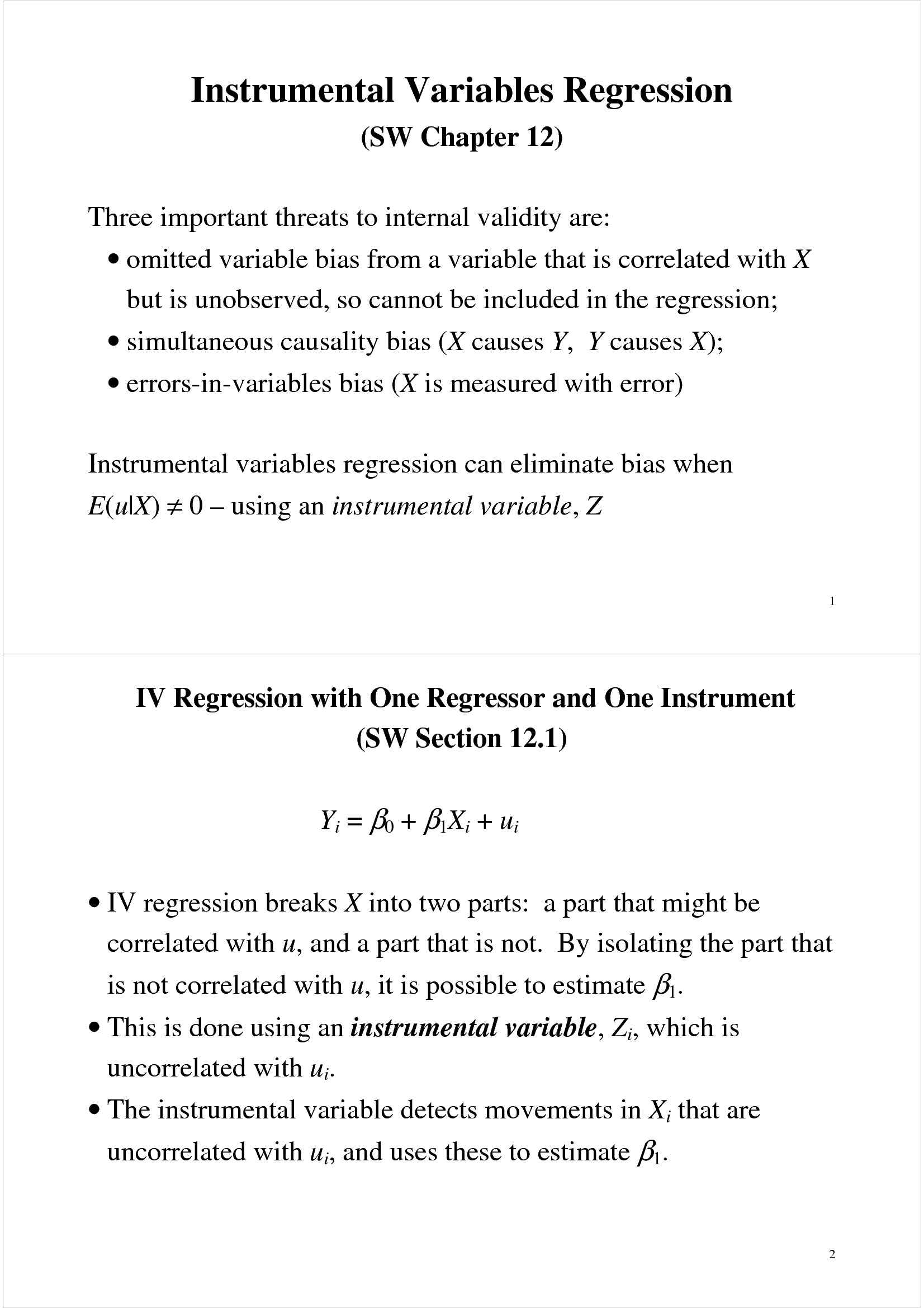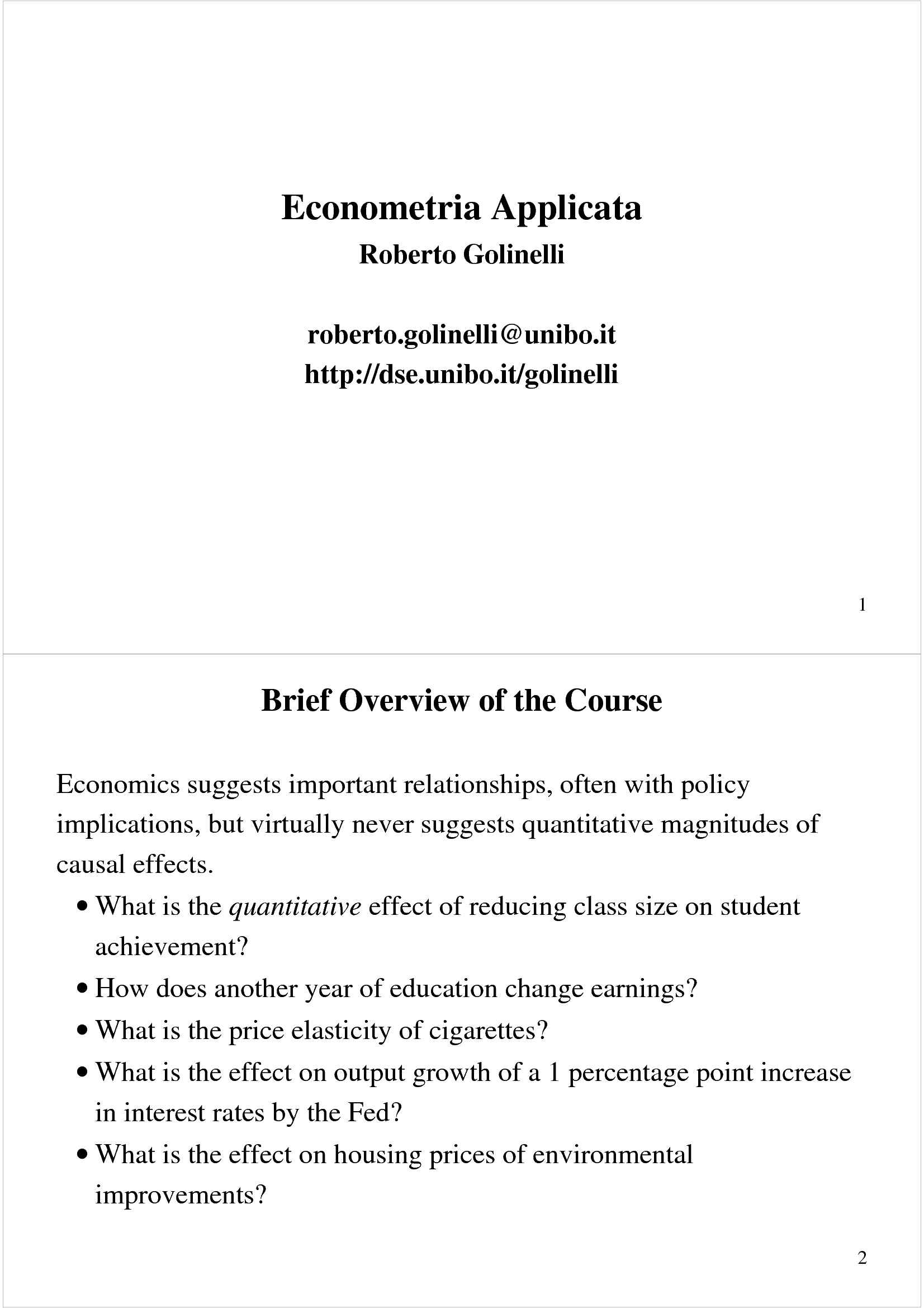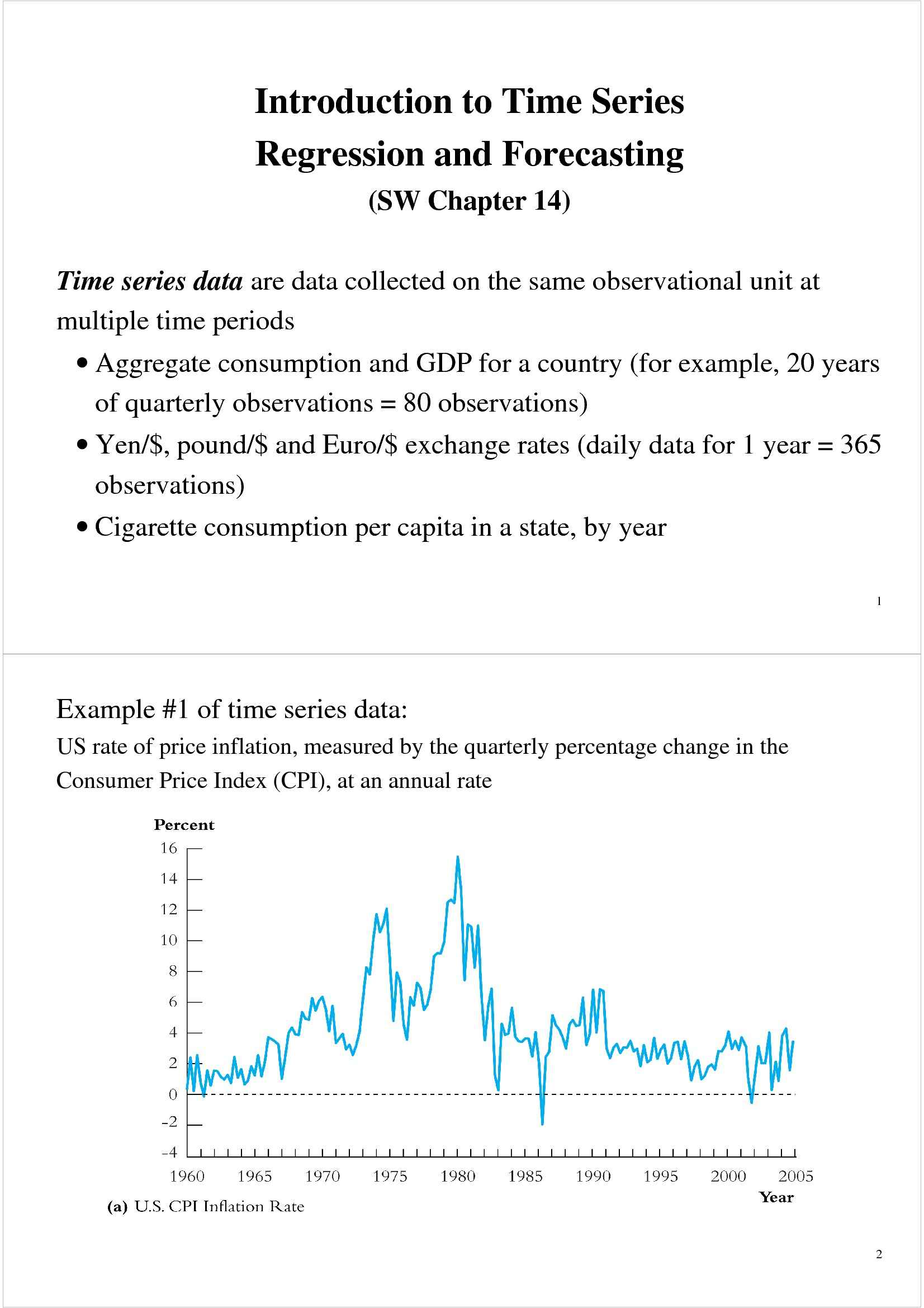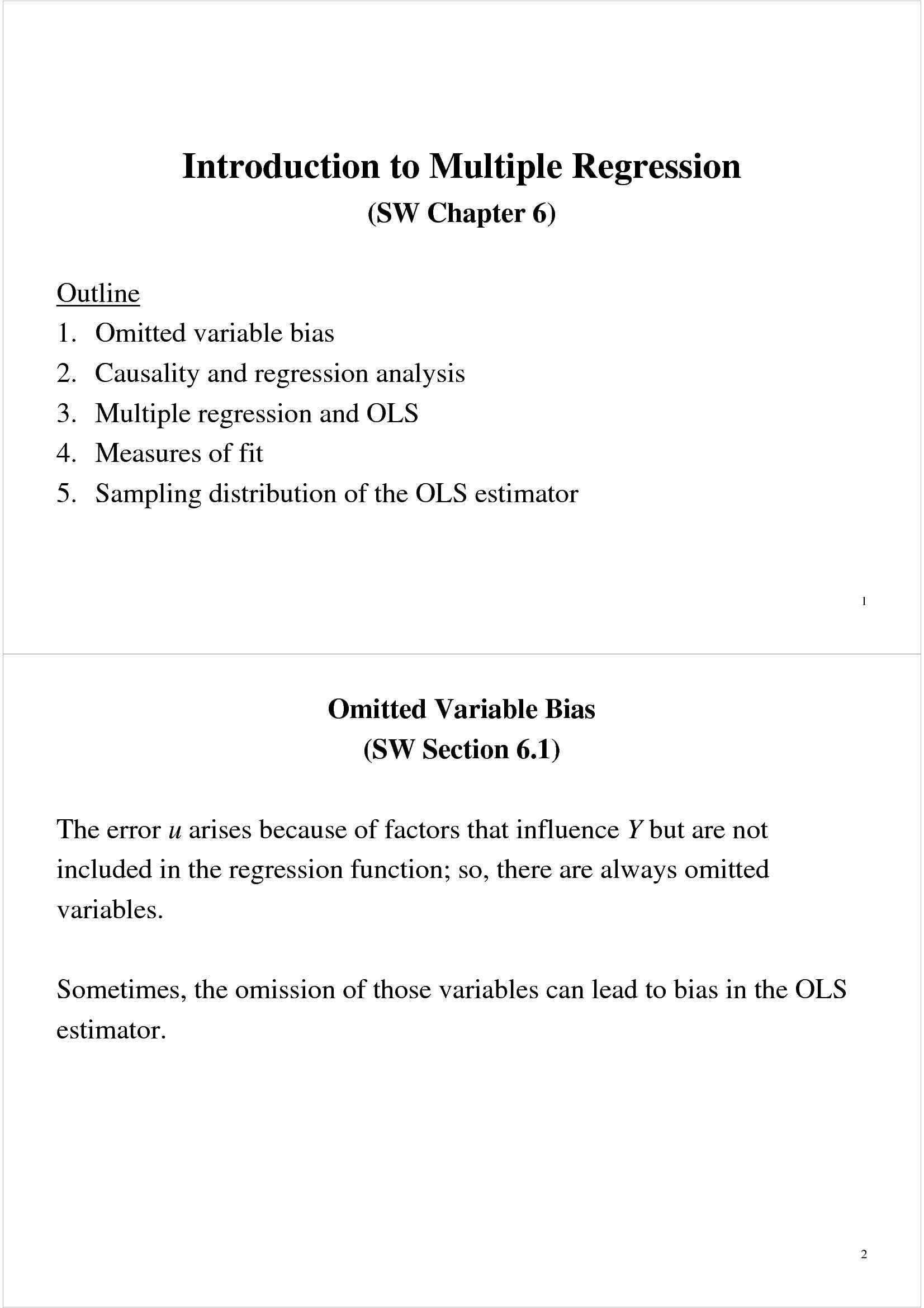Che materia stai cercando?

# Riepilogo di concetti statistici

Materiale didattico per il corso di Econometria applicata del prof. Roberto Golinelli. Trattasi di slides in lingua inglese a cura del docente, all'interno delle quali sono affrontati i seguenti argomenti: le variabili casuali; il Teorema del limite centrale; l'intervallo di confidenza; la distibuzione. Vedi di più

Esame di Econometria applicata docente Prof. R. Golinelli

Anteprima

### ESTRATTO DOCUMENTO

1 Some Facts About Random Variables

Proposition 1 Let X and X be any two random variables with finite expected values,

1 2

then E[X + X ] = E[X ] + E[X ] .

1 2 1 2

Proposition 2 Let X be any random variable with a finite expected value and a, b real

numbers, then E[aX + b] = aE[X] + b

and 2

Var[aX + b] = a Var[X] .

Proposition 3 Let X and X be two independent random variables with finite vari-

1 2

ances, then Var[X + X ] = Var[X ] + Var[X ] .

1 2 1 2

2

∼ 6

Proposition 4 Let X N (µ, σ ) and a, b be two real number with a = 0. Then

2 2

aX + b N (aµ + b, a σ ) . 2

∼ −

Theorem 1 (Standardization Theorem) X N (µ, σ ) if and only if Z = (X

µ)/σ N (0, 1). ∼

Proposition 5 Let X and X be two independent random variables such that X

1 2 1

2

2 ∼ ), then

) and X N (µ , σ

N (µ , σ 2 2

1 2

1 2 2

X + X N (µ + µ , σ + σ )

1 2 1 2 1 2

and 2 2

− ∼ −

X X N (µ µ , σ + σ ) .

1 2 1 2 1 2

2 Central Limit Theorem

Theorem 2 (Central Limit Theorem) Let X , X , . . . , X be independent and iden-

1 2 n 2

tically distributed (iid) random variables with expected value µ and variance σ . If n is

“large”, then the random variable X̄ defined by · · ·

X + X + + X

1 2 n

X̄ = n 2

is approximately normally distributed with an expected value µ and variance σ /n.

Corollaries to the Central Limit Theorem are:

Corollary 1 If X̄ is the sample mean of a random sample (with or without replace-

ment), of size n, take from a infinite population with a mean µ and variance σ, then for

large n (n 30) we have 2

X̄ N (µ, σ /n) .

1

Corollary 2 If X̄ is the sample mean of a random sample with replacement, of size n,

take from a finite population with a mean µ and variance σ, then for large n (n 30)

we have 2

X̄ N (µ, σ /n) .

Corollary 3 If p̂ is the sample proportion of a random sample (with replacement if the

population is of finite size) taken from a population with a population proportion p, then

≥ − ≥

for large n (np 5 and n(1 p) 5) we have

∼ −

p̂ N (p, p(1 p)/n) .

≥ − ≥ ∼ −

Corollary 4 For large n (np 5 and n(1 p) 5) we have Bin(n, p) N (np, np(1

p)).

3 Estimation

A descriptive measure used to describe a population is called a parameter. Therefore a

parameter is a characteristic of a population (like population mean, median, variance,

etc.). A descriptive measure used to describe a sample is called a statistic. Therefore a

statistic is a characteristic of a sample (like sample mean, median, variance, etc.).

An estimator is any method to guess an unknown parameter using sample data.

For example, the sample mean is an estimator of the population mean. Note that an

estimator is a random variable. An estimate is an observed value of an estimator. For

example, if we use the sample median as an estimator for the population median, and

the median of the sample we take is 5, then our estimate for the population median is 5.

In general, if Ω is the parameter to be estimated then we denote an estimator of Ω

with Ω̂. Given a sample data x , x , . . . , x the value Ω̂(x , x , . . . , x ) is an estimate for

1 2 n 1 2 n

Ω. Below we give several estimators for the population mean µ.

1

1 (x + . . . + x )

µ̂ (x , . . . , x ) = 1 n

1 n n

2

µ̂ (x , . . . , x ) = x

1 n 1

1

3 } }

µ̂ (x , . . . , x ) = (min{x , . . . , x + max{x , . . . , x

1 n 1 n 1 n

2

4 {x }

µ̂ (x , . . . , x ) = Median of , x , . . . , x

1 n 1 2 n

Consider a sample consisting of 1, 4, 2, 5, 6 take from a population with unknown mean.

The following table gives, for each of the estimators given above, the estimate based on

the given sample. Estimator Estimate

1

µ̂ 4.2

2

µ̂ 1.0

3

µ̂ 3.5

4

µ̂ 4.0

2

PAGINE

6

PESO

92.47 KB

AUTORE

PUBBLICATO

+1 anno fa

### DESCRIZIONE DISPENSA

Materiale didattico per il corso di Econometria applicata del prof. Roberto Golinelli. Trattasi di slides in lingua inglese a cura del docente, all'interno delle quali sono affrontati i seguenti argomenti: le variabili casuali; il Teorema del limite centrale; l'intervallo di confidenza; la distibuzione.

DETTAGLI
Corso di laurea: Corso di laurea in economia, mercati e istituzioni
SSD:
Università: Bologna - Unibo
A.A.: 2011-2012

I contenuti di questa pagina costituiscono rielaborazioni personali del Publisher Atreyu di informazioni apprese con la frequenza delle lezioni di Econometria applicata e studio autonomo di eventuali libri di riferimento in preparazione dell'esame finale o della tesi. Non devono intendersi come materiale ufficiale dell'università Bologna - Unibo o del prof Golinelli Roberto.

Acquista con carta o conto PayPal

Scarica il file tutte le volte che vuoi

Paga con un conto PayPal per usufruire della garanzia Soddisfatto o rimborsato

RecensioniTi è piaciuto questo appunto? Valutalo!

## Altri appunti di Econometria applicataDispensa

### Regressione con variabili strumentaliDispensa

### Econometria - elementiDispensa

### Regressione forecasting e time seriesDispensa